Publicité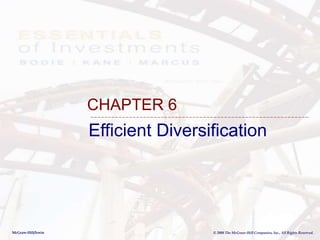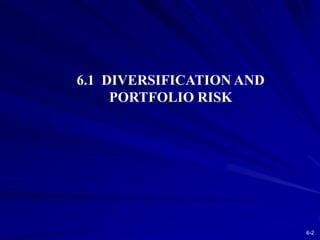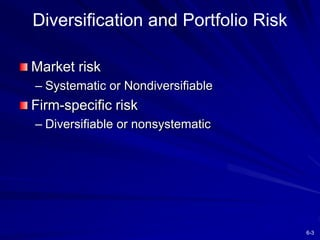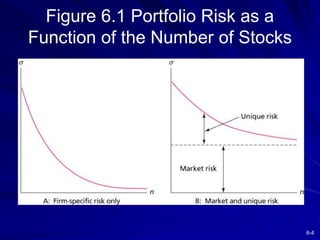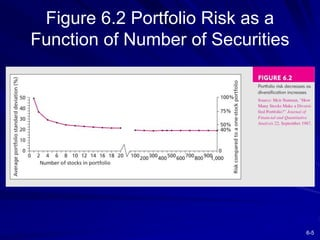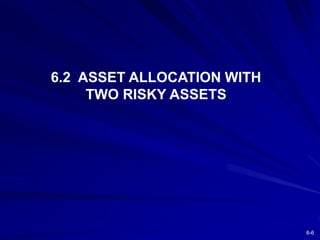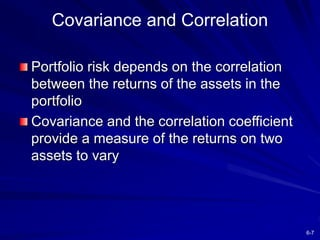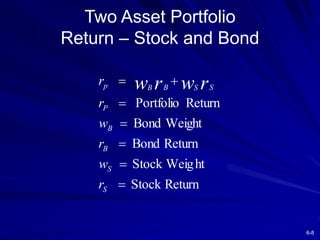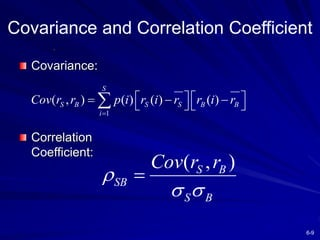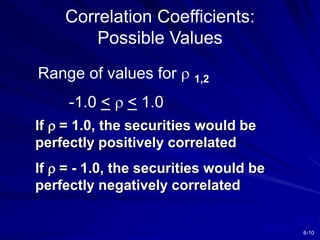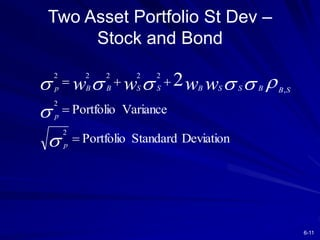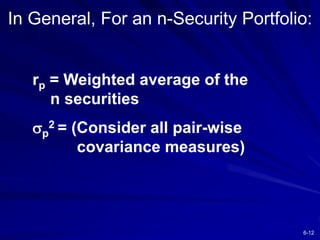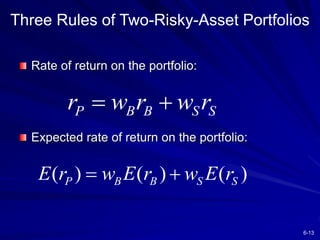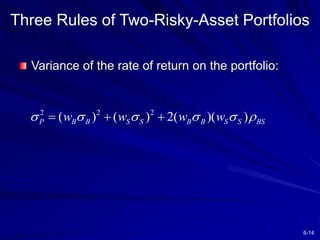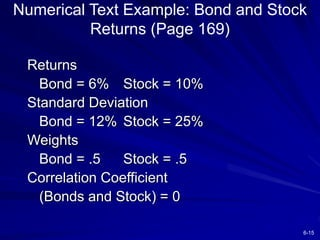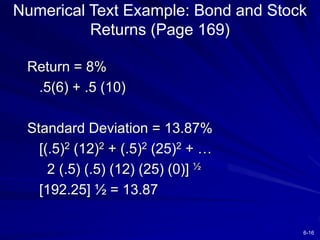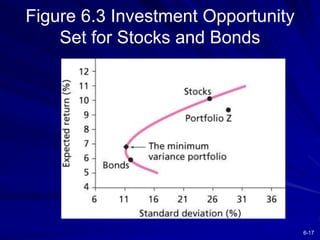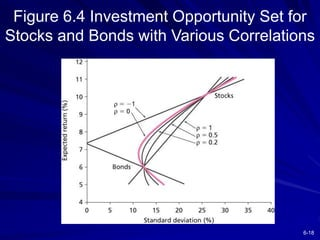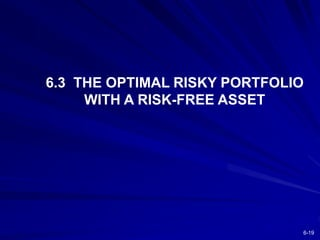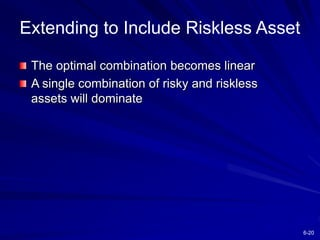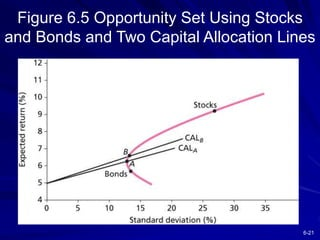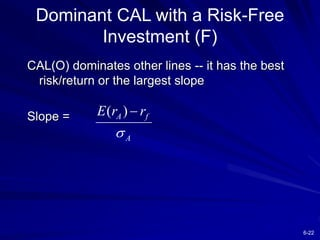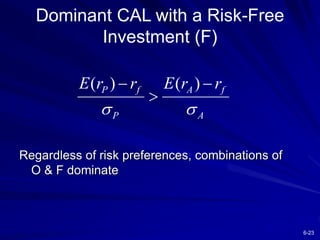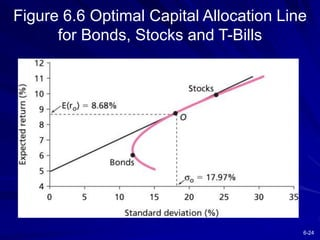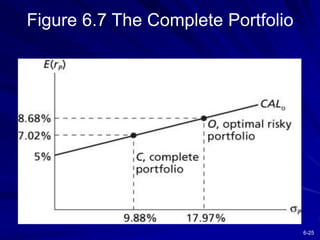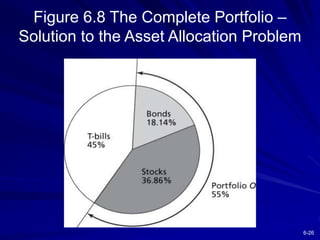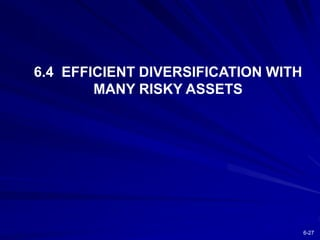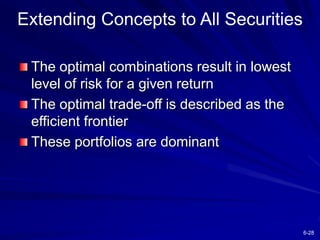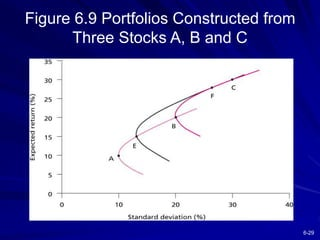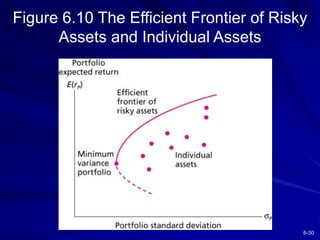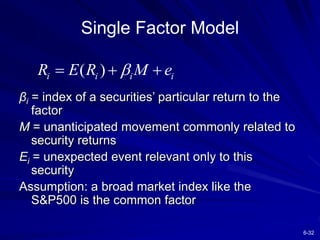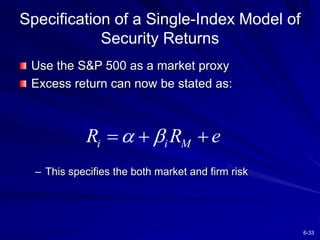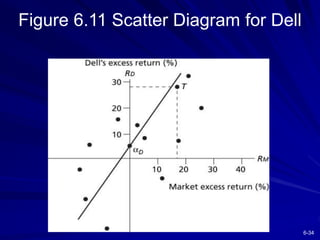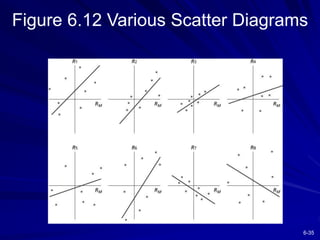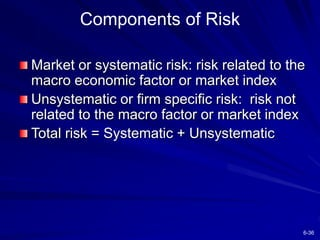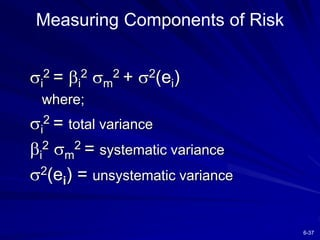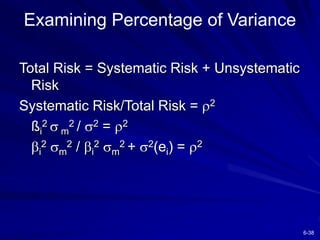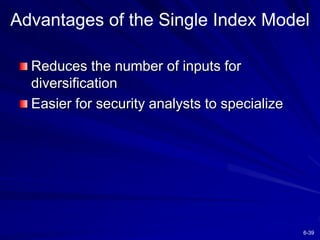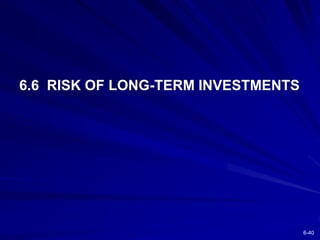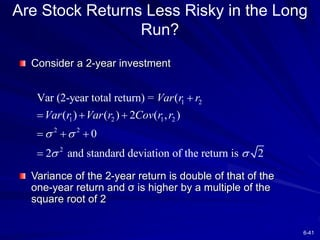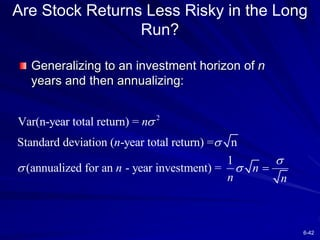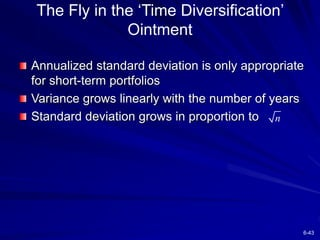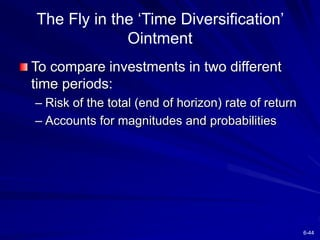1 sur 44
Publicité

### Chapter_006.ppt

2. 6-2 6.1 DIVERSIFICATION AND PORTFOLIO RISK
3. 6-3 Diversification and Portfolio Risk Market risk – Systematic or Nondiversifiable Firm-specific risk – Diversifiable or nonsystematic
4. 6-4 Figure 6.1 Portfolio Risk as a Function of the Number of Stocks
5. 6-5 Figure 6.2 Portfolio Risk as a Function of Number of Securities
6. 6-6 6.2 ASSET ALLOCATION WITH TWO RISKY ASSETS
7. 6-7 Covariance and Correlation Portfolio risk depends on the correlation between the returns of the assets in the portfolio Covariance and the correlation coefficient provide a measure of the returns on two assets to vary
8. 6-8 Two Asset Portfolio Return – Stock and Bond Return Stock ht Stock Weig Return Bond Weight Bond Return Portfolio        S S B B P S S B B p r w r w r r r w r w
9. 6-9 Covariance and Correlation Coefficient Covariance: Correlation Coefficient: 1 ( , ) ( ) ( ) ( ) S S B S S B B i Cov r r p i r i r r i r              ( , ) S B SB S B Cov r r    
10. 6-10 Correlation Coefficients: Possible Values If  = 1.0, the securities would be perfectly positively correlated If  = - 1.0, the securities would be perfectly negatively correlated Range of values for  1,2 -1.0 <  < 1.0
11. 6-11 Two Asset Portfolio St Dev – Stock and Bond Deviation Standard Portfolio Variance Portfolio 2 2 , 2 2 2 2 2 2              p p S B B S S B S S B B p w w w w
12. 6-12 rp = Weighted average of the n securities p 2 = (Consider all pair-wise covariance measures) In General, For an n-Security Portfolio:
13. 6-13 Three Rules of Two-Risky-Asset Portfolios Rate of return on the portfolio: Expected rate of return on the portfolio: P B B S S r w r w r   ( ) ( ) ( ) P B B S S E r w E r w E r  
14. 6-14 Three Rules of Two-Risky-Asset Portfolios Variance of the rate of return on the portfolio: 2 2 2 ( ) ( ) 2( )( ) P B B S S B B S S BS w w w w         
15. 6-15 Numerical Text Example: Bond and Stock Returns (Page 169) Returns Bond = 6% Stock = 10% Standard Deviation Bond = 12% Stock = 25% Weights Bond = .5 Stock = .5 Correlation Coefficient (Bonds and Stock) = 0
16. 6-16 Numerical Text Example: Bond and Stock Returns (Page 169) Return = 8% .5(6) + .5 (10) Standard Deviation = 13.87% [(.5)2 (12)2 + (.5)2 (25)2 + … 2 (.5) (.5) (12) (25) (0)] ½ [192.25] ½ = 13.87
17. 6-17 Figure 6.3 Investment Opportunity Set for Stocks and Bonds
18. 6-18 Figure 6.4 Investment Opportunity Set for Stocks and Bonds with Various Correlations
19. 6-19 6.3 THE OPTIMAL RISKY PORTFOLIO WITH A RISK-FREE ASSET
20. 6-20 Extending to Include Riskless Asset The optimal combination becomes linear A single combination of risky and riskless assets will dominate
21. 6-21 Figure 6.5 Opportunity Set Using Stocks and Bonds and Two Capital Allocation Lines
22. 6-22 Dominant CAL with a Risk-Free Investment (F) CAL(O) dominates other lines -- it has the best risk/return or the largest slope Slope = ( ) A f A E r r  
23. 6-23 Dominant CAL with a Risk-Free Investment (F) Regardless of risk preferences, combinations of O & F dominate ( ) ( ) P f A f P A E r r E r r     
24. 6-24 Figure 6.6 Optimal Capital Allocation Line for Bonds, Stocks and T-Bills
25. 6-25 Figure 6.7 The Complete Portfolio
26. 6-26 Figure 6.8 The Complete Portfolio – Solution to the Asset Allocation Problem
27. 6-27 6.4 EFFICIENT DIVERSIFICATION WITH MANY RISKY ASSETS
28. 6-28 Extending Concepts to All Securities The optimal combinations result in lowest level of risk for a given return The optimal trade-off is described as the efficient frontier These portfolios are dominant
29. 6-29 Figure 6.9 Portfolios Constructed from Three Stocks A, B and C
30. 6-30 Figure 6.10 The Efficient Frontier of Risky Assets and Individual Assets
31. 6-31 6.5 A SINGLE-FACTOR ASSET MARKET
32. 6-32 Single Factor Model βi = index of a securities’ particular return to the factor M = unanticipated movement commonly related to security returns Ei = unexpected event relevant only to this security Assumption: a broad market index like the S&P500 is the common factor ( ) i i i i R E R M e    
33. 6-33 Specification of a Single-Index Model of Security Returns Use the S&P 500 as a market proxy Excess return can now be stated as: – This specifies the both market and firm risk i i M R R e     
34. 6-34 Figure 6.11 Scatter Diagram for Dell
35. 6-35 Figure 6.12 Various Scatter Diagrams
36. 6-36 Components of Risk Market or systematic risk: risk related to the macro economic factor or market index Unsystematic or firm specific risk: risk not related to the macro factor or market index Total risk = Systematic + Unsystematic
37. 6-37 Measuring Components of Risk i 2 = i 2 m 2 + 2(ei) where; i 2 = total variance i 2 m 2 = systematic variance 2(ei) = unsystematic variance
38. 6-38 Total Risk = Systematic Risk + Unsystematic Risk Systematic Risk/Total Risk = 2 ßi 2  m 2 / 2 = 2 i 2 m 2 / i 2 m 2 + 2(ei) = 2 Examining Percentage of Variance
39. 6-39 Advantages of the Single Index Model Reduces the number of inputs for diversification Easier for security analysts to specialize
40. 6-40 6.6 RISK OF LONG-TERM INVESTMENTS
41. 6-41 Are Stock Returns Less Risky in the Long Run? Consider a 2-year investment Variance of the 2-year return is double of that of the one-year return and σ is higher by a multiple of the square root of 2 1 2 1 2 1 2 2 2 2 Var (2-year total return) = ( ( ) ( ) 2 ( , ) 0 2 and standard deviation of the return is 2 Var r r Var r Var r Cov r r            
42. 6-42 Are Stock Returns Less Risky in the Long Run? Generalizing to an investment horizon of n years and then annualizing: 2 Var(n-year total return) = Standard deviation ( -year total return) = n 1 (annualized for an - year investment) = n n n n n n      
43. 6-43 The Fly in the ‘Time Diversification’ Ointment Annualized standard deviation is only appropriate for short-term portfolios Variance grows linearly with the number of years Standard deviation grows in proportion to n
44. 6-44 The Fly in the ‘Time Diversification’ Ointment To compare investments in two different time periods: – Risk of the total (end of horizon) rate of return – Accounts for magnitudes and probabilities
Publicité